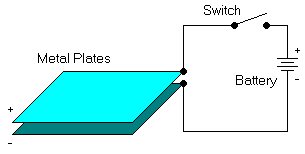# Capacitors

What is a capacitor? Why do we use them in a coilgun? How can you put some little capacitors together to make a big capacitor?

## Background on CapacitorsA capacitor is an electrical device that has, in DC circuits, the purpose of storing energy. In particular, it stores an electrical charge.

Suppose two flat metal plates are placed close to each other (but not touching) and are connected to a battery through a switch. At the instant the switch is closed, electrons are attracted from the upper plate to the positive terminal of the battery, and the same number are repelled into the lower plate from the negative battery terminal. Enough electrons move into one plate and out of the other to make the voltage between them the same as the voltage of the battery.

If the switch is opened after the plates have been charged in this way, the top plate is left with a deficiency of electrons and the bottom plate with an excess. Since there is no current path between the two, the plates remain charged despite the fact that the battery no longer is connected.

You can do a simple experiment with a large capacitor, such as a large electrolytic capacitor from a power supply (or a coilgun!). Charge it up to ten or twenty volts. Disconnect the power. When you measure the voltage on the capacitor's terminals, you find it may remain for a very long time, perhaps even days or months! I have been shocked (literally) to find a capacitor in a surplus parts store that spit sparks when I shorted the terminals together, and who knows how many months it had been sitting there!

## Energy Storage

The charge or quantity of electricity that can be held in the electric field between the capacitor plates is proportional to the applied voltage and to the capacitance of the capacitor: Q = C * V where

• Q = charge in coloumbs
• C = capacitance in farads
• V = voltage in volts

The energy stored in a capacitor is also a function of voltage and capacitance: W = V2 * C / 2 where

• W = energy in joules (watt-seconds)
• V = voltage in volts
• C = capacitance in farads

## A Crash Course on Timing

A capacitor stores energy, and we can use it to make a quick and powerful electric discharge to propel our projectile. Why not use a large battery? Because the internal resistance of a battery is much higher than a capacitor. It takes a much longer time to get the same amount of energy from a battery, and we will see that timing is very important. In fact, using a different combination of capacitors can give us the exact control we need over this delicate issue.

Charging capacitors is really not an issue... Just insert them in a circuit with a voltage that does not exceed the capacitor's voltage rating. Wait till current stops flowing, and your caps are fully charged.

Discharge time is the real trick! Here's what we are trying to do: Have as much current as possible until the projectile is halfway down the tube. Anything longer than that is not just a waste of energy, it actually hurts performance! If some current is still in the coil when the projectile goes past the middle it will actually PULL THE PROJECTILE BACKWARDS. (In highly technical terms this is known as "the suckback effect".) Thus it will at least slow down, if not pulling it back into the coil. On the other side, if current dies down before the projectile is halfway through the coil it will reduce efficiency. If you have to go for a comprise, be sure to opt for the second choice though.

Experiment to find what works best for you, and let us know!

 < Previous Page 1 of 6 Next >